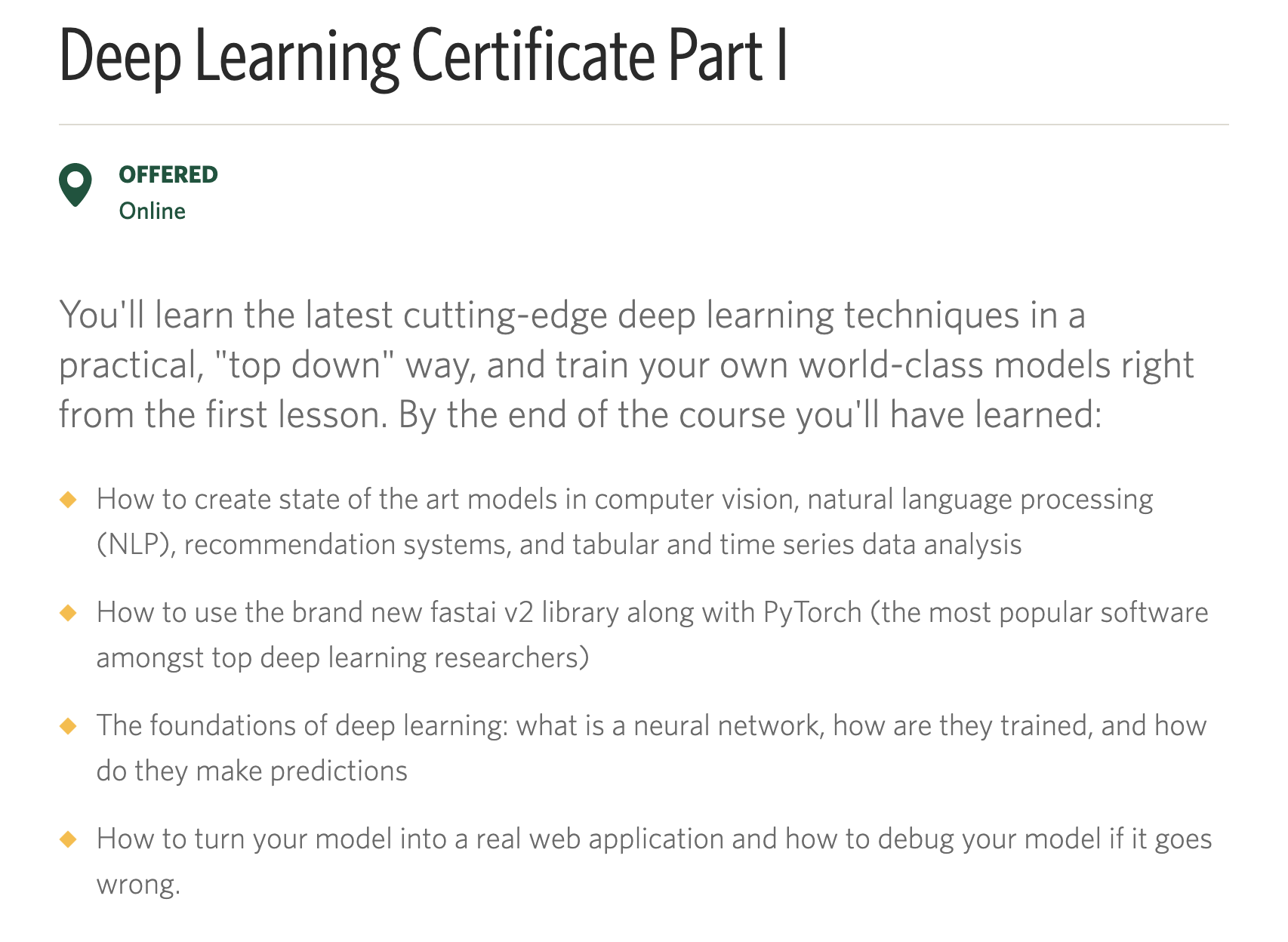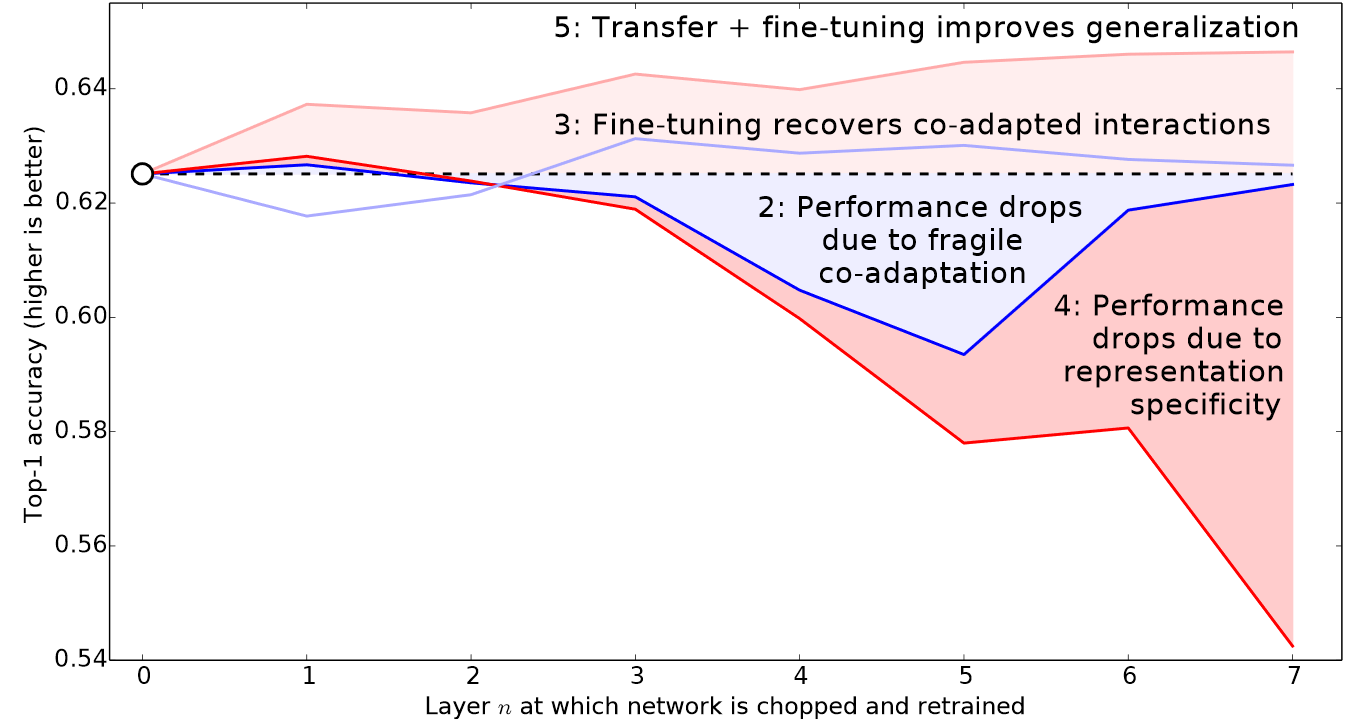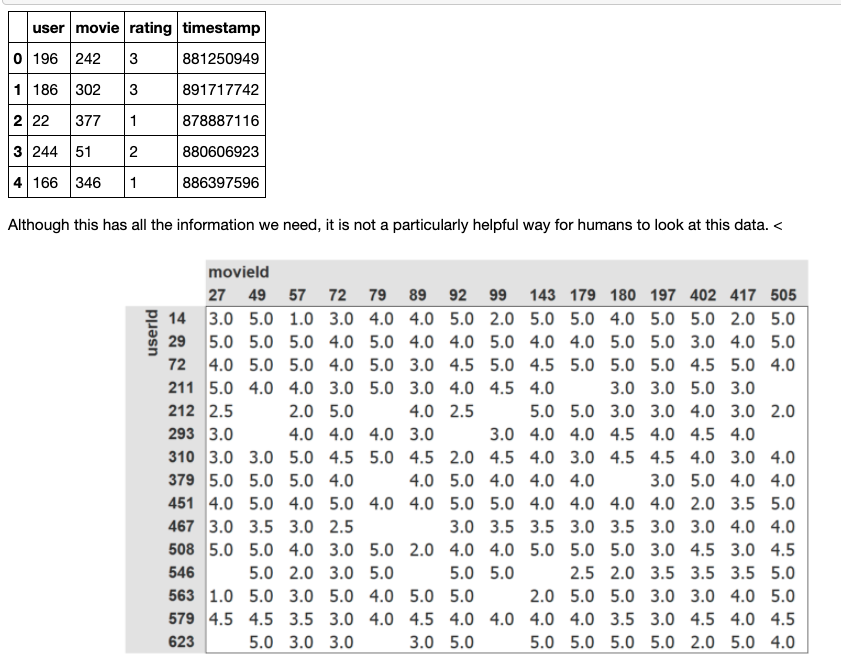# Part1 lesson6 note | fastai 2020 course-v4

Apr 23, 2020 · 14 mins readShare this

Note of lesson6 : Deep Learning Part 1 of Spring 2020 at USF

Today we will study

1. Image Classification
2. Multi-label classification
3. Collaborative filtering

## CONTENTS

Previous; Recap of last class

## Image Classification

• When you have many categories, use most_confused() method rather than plotting confusion matrix 1

### Improving our model

#### Learning rate finder

• We can try to learn fast which is done by epoch 1~2 and set learning rate big
• learn.fine_tune() has base_lr parameter
• If learning rate is too high, loss validation gets too high compared to loss of train
• why? because it diverges
• If learning rate is too small, train loss decreases too slowly, and there are much gap between train and valid loss. 2
• So it will take long time, means too many epochs, resulting overfitting
• meaning of learning rate ~ loss graph
• find optimal lr drawing loss depends on learning rate
• careful it’s logarithm scale
• this method was invented at 15 and before that people just experimented

lr_find() goes through one sing mini-batch? J: no, it’s just working through data-loader, and diff is we try many lrs

why steepest? why not minimum? J: at the minimum we don’t learn anymore. we want our model to enhance learning while training
R: About lr_find(), it’s natural that it seems too simple

#### Unfreezing and transfer learning

• we throw away last layer, and re-train depends on our data/task
• And the below function is easy way when we do just fine-tune
learn.find_tune??

• calling cnn_learner freeze our model, so don’t have to do it separately
• But when you do unfreeze, which means you train all the params, you need to adjust the learning rate more.
• We can do better because we just used the same learning rate for a whole training

#### Discriminative learning rates• use slice at lr_max parameter means discriminative learning rate 3

#### Selecting the number of epochs

• If you choose too big epoch, valid_loss will not change from specific point, and this is related to fit_one_cycle

• What fit_one_cycle does?
• different from just fit.
• start purposely from low learning rate. and from the point of highest point, they decrease lr again
• if error_rate stop from specific epoch, use that epoch as epoch and do it again
• Loss is just a thing we want to approximate, so always care error

#### Deeper architectures

• It would be possible that you don’t want pre-trained but usually it’s helpful
• usually out of memory happens
• about, .to_fp16(): half precision floating point, NVIDIA GPU support special tensor and it’s faster about 2x/3x
• you can use this using module from fastai2.callback.fp16 import *
• Try a small model before scaling up the model < because bigger model doesn’t guarantee better performance

### Assignment

• Don’t forget the questionnaire
• Read paper, Cyclical Learning Rates for Training Neural Networks and see how you can get best results with adjusting learning rate, find out best learning rate by yourself!
• of course you can see fast.ai learning rate source code

How do you know you can do better? J: you don’t know, who knows. just try and experiment

about .to_fp16() it doesn’t affect result? J: less bumpy, little bit better but not that big deal

question about random J: not does machine learning but deep learning specifically. For more info, see Rachael’s Linear Algebra course.

## Multi-label classification

### Constructing a data block

• pandas, which is for dataframe
• when you slicing, : is always optional
• Read Python for Data Analysis class written by person who made pandas
• dataset is abstract idea of class
• If you get index, and length, it’s dataset.
• string.ascii_lowercase is qualified dataset since it has length and index
• If you index it mostly get tuple, independent variable and dependent variable.
• Datasets is usually composed of not stack of data and label
• fast.ai, we implemented use file name as independent variable, and through function we get dependent variable
• Dataloader divides Datasets to batches, read the book

• Datablock assumes basically we have 2 kind of variable
• randomly select different validation set so we have different output whenever we call dataset
dblock = DataBlock(blocks=(ImageBlock, MultiCategoryBlock),
get_x = get_x, get_y = get_y)
dsets = dblock.datasets(df)
dsets.train


 (PILImage mode=RGB size=500x375, TensorMultiCategory([0., 0., 0., 0., 0., 0., 0., 0., 0., 0., 0., 1., 0., 0., 0., 0., 0., 0., 0., 0.]))

• PILImage mode=RGB size=500x375: independent variable, which is a input
• we did transform filename to tensors
• output is one-hot encoding

Shouldn’t it be an integer but why output(TensorMultiCategory([0., 0., 0., 0., 0., 0., 0., 0., 0., 0., 0., 1., 0., 0., 0., 0., 0., 0., 0., 0.]))) is float? J: because we will use cross-entropy style loss, which does floating calculation

why we don’t use with floating point 8 bit? J: could be fast, but low precision (but maybe possible)

#### Binary cross entropy

learn = cnn_learner(dls, resnet18)
x,y = dls.train.one_batch()
activs = learn.model(x)
activs.shape

• you can try grapping the one batch of the data to the model, you can get an output.
• Use adls.train.one_batch() function to ensure what’s going in and out
def binary_cross_entropy(inputs, targets):
inputs = inputs.sigmoid()
return -torch.where(targets==1, inputs, 1-inputs).log().mean()

• result is 0 to 1 but we get a variable between 0 and 1 so that we use sigmoid
• same reason as we said at softmax, normally we use log  (fast and acurate)

• explained the book

• One-hot encoded target, use BCELoss
• accuracy as a metric only works for single label dataset
• because accuracy does argmax which chooses largest number
• so doing multi-categorical problem, use metrics which can compare each activation with some threshold(not selecting one value), and pass if the number over threshold
• but we might want to change threshold depends on the input, so that we use partial function which sets default variable, and change if we want
learn = cnn_learner(dls, resnet50, metrics=partial(accuracy_multi, thresh=0.2))

• the accuracy changes if I use different threshold
• How can I get best threshold?
preds,targs = learn.get_preds()
xs = torch.linspace(0.05,0.95,29)
accs = [accuracy_multi(preds, targs, thresh=i, sigmoid=False) for i in xs]
plt.plot(xs,accs);

• we can plot and select the best number and don’t have to care of overfitting (because it’s not that bumpy as rule of thumb)

### Regression

• two types of task at supervised learning 1) classification(discrete variable) 2) regression(continuous variable)

#### Assemble the data

• get_image_files gather image recursively
• here, it important to setting evaluation to a specific person usingsplitter, not randomly selection -> it’s continuous frame, so if you just select random data, it would be overestimated.
• pytorch has tensor batch, channel, height, width
• regarding channel, image has 3 channels
• you can see the version of R, G, B using the below code
im = image2tensor(Image.open('image/grizzly.jpg'))
_, axs = subplots(1,3)
for bear, ax, color in zip(im, axs, ('Reds', 'Greens', 'Blues')):
show_images(255-bear, ax=ax, cmap=color)


can we give multi-channel bigger than 3? J: yes, it used often like when you use satellite image. But your pre-trained model is usually for a 3-channel and fastai handles that case, but is is just problem of axis

#### Training a mode

learn.show_results(ds_idx=1, max_n=3, figsize=(6,8))

• left of output is target, right is prediction
• Notice It’s interesting that basically our pre-trained model was trained for classification task. But it works well when you do regression
• why? pre-trained model from ImageNet is collection of image’s features like, objects, color, texture, shadow and so on
• so from pre-trained model, we can get the features of an image, and do the other job(e.g., regression) with fine-tuning

### Assignment

• go to the bear classifier, and find out when you give image which is not included in label and see what happens. change model from single labelling to multi-labelling, and tell what happened at fastai forum

at traditional machine learning we used cross-validation, we don’t use it at deep learning? J: First, nowadays cross-validations are less common(if not on kaggle, because praction of data is important), because cross-validation was used there were not lots of data. Second, cross-validation is not related selection between machine learning and deep learning

CF algorithm used other than recommendation system J: whatever it’s kind of recommendation system, if concept is finding out the other candidates using the previous behaviour

how can i make data set if I have video? how split?(time phrase) J: like full color movie, rank-5 tensor by (time, h, w, batch, channel). but usually it would be 4 tensor, because of size of tensor or key problem, but theoretically possible.

## Collaborative filtering

• empty part means user didn’t watch or rate the movie
• upper and below part represent same info### Learning the latent factors

• CF algorithm is related to latent variable(;factors) since we don’t have a label explaining/depicting properties which made you choose specific movie
• In other words, there is no specific label how we could predict the dependent variable(recommendation; movies user didn’t watch) results from independent var(rating regarding user and movie)
• but we can assume it has labels like genres and make matrix representing it
• randomly create user’s factor(;latent, hidden) and movie’s factor. and do matrix multiplication with those, which is dot product.
• and sum it and compare with target, so that you can learn parameters, which were randomly initialized

### Creating the DataLoaders

• Let’s make DataLoaders using basic python and PyTorch!

• user and movie is represented using index, label,..,called look up in an index
• but this is problem, because DL treats only kind of matrix multiplication.
• so that we make one-hot encoding matrix
• And this is quite amazing because it came out with any kind of discrete value, we can make it work using arrays

how is different when you treat actual data between dense and sparse data? J: we will not treat sparse data, but there’s course Rachael’s linear algebra

• embedding layer: multiplying by a one hot encoded matrix, using the computational shortcut that it can be implemented by simply indexing directly.
• there is excel explaining detailed computation
• This is important because usually people think embedding is something difficult, but it’s just computational shortcut to do matrix multiplication

### Collaborative filtering from scratch

• importance of dunder method of python. (like __init__)
• explained OOP concept
• Inheritance of python, which used DotProduct class
• Module is fast.ai version of pytorch’s nn.Module
• forwardfunction grab embedding, using specific index
• (again) Remember Embedding is just kind of shortcut to make a one-hot encoding matrix
class DotProduct(Module):
def __init__(self, n_users, n_movies, n_factors):
self.user_factors = Embedding(n_users, n_factors)
self.movie_factors = Embedding(n_movies, n_factors)

def forward(self, x):
users = self.user_factors(x[:,0])
movies = self.movie_factors(x[:,1])
return (users * movies).sum(dim=1)

• 64: size of mini_batch, 2: index
x,y = dls.one_batch()
x.shape


torch.Size([64, 2])

• We use bias to represent specific character of each user and movie, because embedding of user/movie doesn’t show users’ character, who might generally doesn’t like movie

• what should we do when my model is overfitting very quickly with small epoch

• i.e., what should we do to evade overfitting and don’t want to lessen epoch?
• augmentation can be one solution but we can’t do augmentation with this data
• in this case, we do other regularization, which means panellize the fast learning 4

Collaborative filtering algorithm works better than svd or kind of that? J: yes

My Questions

1. no way to see with regression model?

2. But how do I know if loss decreases slowly or not?

3. I need to study more of scheduled learning

4. Isn’t there any side effects when you did too much regularization?